#### Number system and codes, Physics tutorial

Binary number system:

Consider familiar decimal system. In this system there are 10 distinct and different digits (0, 1, 2, 3, 4, 5, 6, 7, 8, and 9). For magnitudes greater than 9, convention is to arrange digits in rows starting with most significant on left and concluding with least significant on right. Significance is determined by what is known as weighting of digit.

Therefore concept of tens, hundreds, thousands, etc arises. For instance 3458 = (3x103) + (4x102) + (5x101) + (8x100). Each digit is one of the symbols 0 to 9 and is multiplied by the power of ten, depending on position of digit. Therefore, decimal numbers are said to have base of ten and multiplying powers 100, 101, 102,103, etc. are known as weight or positional values.

In binary number system (base of 2), there are only two digits: 0 and 1 and place values are 20, 21, 22, 23 etc. Binary digits are abbreviated as bits. For instance 1101 is binary number of 4 bits (i.e., it is binary number containing four binary digits.)

The binary number may have any number of bits. Consider number 11001.011. Note binary point in this number. The bit on extreme right is known as least significant bit (LSB) and bit on extreme left is known as most significant bit (MSB). Each bit has its positional value as shown below:

24  23  22  21  20     2-1  2-2  2-3  Positional values or weight

1   1   0    0    1   .   0   1    1

↑                       ↑             ↑

MSB        Binary point      LSB

Bits on left of binary point are positive powers of 2 and bits on right of binary point are negative powers of 2. The decimal equivalent of this number is found by summing the products of each bit and its positional value as follows:

11001.0112 = (1x24) + (1x23) + (0 x 22) + (0 x 21) + (1 x 20) + (0 x -1) + (1 x 2-2) + (1 x 2-3)

= 16 + 8 + 0 + 0+1+0 + 0.250 + 0.125 = 25.37510.

The benefit of binary system is that it has made job of designing digital circuitry very simple as only two different states or levels of voltages have to be managed. For instance ON state of the bulb may be signified by bit 1 and OFF state by 0. In terms of voltages, 0 V or a LOW voltage may signify bit 0 and 5V or a HIGH voltage may represent bit 1. Actually, it is not essential also to have precise voltages allotted to each bit. In analogue system exact value of voltage is very significant that makes design of accurate analogue circuitry very difficult. Though, in digital systems exact value of voltage is not significant because voltage of 3.9 V means same thing as voltage of 4.4 V or 5 V.

Binary to Decimal Conversion:

A binary number can be converted into its decimal equivalent by just adding weights of different positions in binary number that have bit 1. For instance, consider conversion of 100011.1012.

1        0     0      0       1      1.     1     0    1

25  +  0 +  0  +  0  +  21  + 20 + 2-1 + 0 + 2-3

= 32 + 2 + 1 + 0.5 + 0.125 = 35.62510

Consider another example of conversion of 11100111.01013.

1        1       1        0      0       1        1       1.      0      1         0      1

27  +  26  +  25  +  0  +  0  +  22  +  21  +  20  +  0  +  2-2  +  0  +  2-4

= 128 + 64 + 32 + 4 + 2 + 1 + 0.250 + 0.0625 = 231.312510.

Now consider the following examples.

111.100 = 7.5

11.1100 = 3.75

1.11100= 1.875

From given examples it is apparent that if binary point is shifted towards left side, then value of number is halved.

Decimal to Binary Conversion:

The decimal number is converted in its binary equivalent by its repeated divisions by 2. Division is continued till we get the quotient of 0. Then all remainders are set in sequence with first remainder taking position of LSB and last one taking position of MSB. Let conversion of 27 in its binary equivalent. If number also has some figures on right of decimal point, then this part of number is to be treated individually. Multiply this part repetitively by 2. After first multiplication by 2, either 1 or 0 will appear on left of decimal point. Keep this 1 or 0 separately and don't multiply it by 2 subsequently. This must be followed for every multiplication. Carry on multiplication by 2 till you get all 0s after decimal point or up to level of accuracy desired. Consider conversion of 27.62510 in binary equivalent.

As 27 already converted into binary equivalents that is 110112. Now for conversion of 0.625, multiply it by 2 repeatedly as follows: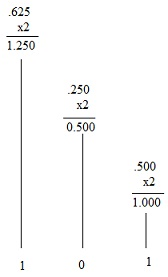Representing numbers in binary is very tiresome as binary numbers frequently comprise of large chain of 0's and 1's. Imagine length of binary equivalent of 10 digit decimal number. So, suitable shorthand forms for representing binary numbers are developed like octal system and hexadecimal system. With such number systems long strings of 0's and 1's can be decreased to manageable form.

Octal number system:

Octal number system has base-8 that is there are 8 digits in this system. These digits are 0, 1, 2, 3, 4, 5, 6, and 7. Weight of each octal digit is some power of 8 depending on position of digit.

84    83    82    81   80     8-1     8-2    Weights

1     0     6      2     7   .   4      5      Octal Number

↑                          ↑          ↑

MSB            Octal point   LSB

Octal number doesn't comprise decimal digits 8 and 9. If any number comprises decimal digits 8and 9, then number can't be octal number.

In octal counting, if n is number of digits then total number of counts is 8n. Largest decimal number represented by the octal number having n digits is 8n-1. Therefore with n = 4, total number of counts is 84 = 4096 and largest decimal number represented is 4096 - 1 = 409510.

Octal to Decimal Conversion:

An octal number can be converted in its decimal equivalent by multiplying octal digit by positional value. For instance,

126.258 = (1 x 83) + (2 x 81) + (6 x 80) + (2 x 8-1) + (5 x 8-2)

= 64 + 16 + 6 + 0.25 + 0.078 = 86.32810

Now convert 36.48 in decimal number.

36.48 = 3 x 81 + 6 x 80 + 4 x 8-1

= 24 + 6 + 0.5 = 30.510

Decimal to Octal Conversion:

The decimal number can be converted by repeated division by 8 in equivalent octal number. This method is like that adopted in decimal to binary conversion. If decimal number has some digits on right of decimal point, then this part of number is converted in its octal equivalent by repeatedly multiplying it by 8. The procedure is same as has been followed in binary number system. Consider conversion of 126.3810 in decimal equivalent. Divide it in two parts that is 126 and .38.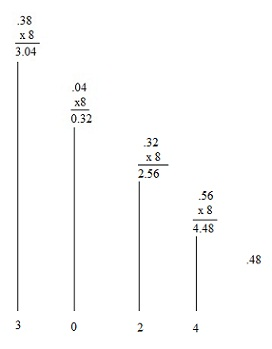Therefore 126.3810 = 176.30248

Octal to Binary Conversion:

In octal number system the highest octal digit, i.e., 7 can be stated as a 3-bit binary number. Therefore, all octal digits have to be represented by the 3-bit binary number. Main benefit of octal number system is easiness with which any octal number can be converted in its binary equivalent.

Using this conversion of octal digit in 3-bit binary number, any octal number can be converted in its binary equivalent by simply replacing each octal digit by a 3-bit binary number. For instance, conversion of 5678 in its binary equivalent is:

5678 = 101 110 111

= 1011101112

Thus 5678 = 1011101112

Binary to Octal Conversion:

The binary number can be converted in its octal equivalent by first making groups of 3-bits starting from LSB side. If MSB side doesn't have 3 bits, then add 0s to make last group of 3 bits. Then by replacing each group of 3 bits by octal equivalent, binary number can be converted in its binary equivalent. For instance, consider conversion of 11000110012 into its octal equivalent as follows:

11000110012 = 1   100   011   001

[As the MSB side does not have 3 bits, we have added two 0's to make the last group of 3 bits]

= 001   100   011   001

= 1           4       3        1

Therefore 11000110012 = 14318

Hexadecimal number system has base-16 that is it has 16 digits (Hexadecimal means 16). The digits are 0, 1, 2, 3, 4, 5, 6, 7, 8, 9, A, B, C, D, E, and F. The digits A, B, C, D, E, and F have equivalent decimal values 10, 11, 12, 13, 14, and 15 respectively. Each Hex (hexadecimal is popularly called as hex) digit in hex number has positional value that is some power of 16 depending on position in number.

164   163   162   161   160     16-1   16-2 Weights

1       2       4      A        F   .    B         9 Hex number

↑                                      ↑             ↑

MSB                         Binary point     LSB

Hex to Decimal Conversion:

Hex to decimal conversion is done in same way as in cases of binary and octal to decimal conversions. Hex number is converted in its equivalent decimal number by summing products of weights of each digit and their values. This is clear from example of conversion of 514.AF16 in decimal equivalent.

514.AF16 = 5x162 + 1x161 + 4x160 + 10x16- 1 + 15x16-2

= 1280 + 16 + 4 + 0.625 + 0.0586

= 1300.683610

Another example:

3BE.1A16 = 3x162 + 11x161 + 14x160 + 1x16- 1 + 10x16- 2

= 768 + 176 + 14 + 0.0625 + 0.0391

= 958.101610

Decimal to Hex Conversion:

The decimal number is converted in hex number in same way as decimal number is converted in its equivalent binary and octal numbers. Part of the number on left of decimal point is to be divided repetitively by 16 and part on right of decimal point is to be repeatedly multiplied by 16. This will be clear from examples of conversion of 579.2610 in hex equivalent. Divide number into two parts 579 and .26.

Therefore 57910 = 24316.

Now .26 is converted into hex number as follows: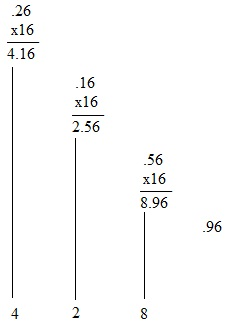Hex to Binary Conversion:

As in octal number system, hex number is converted in its binary equivalent by replacing each hex digit by equivalent 4-bit binary number. This is clear from following example:

BA616 = B A 6

= 1011   1010   0110

= 1011101001102

Binary to Hex Conversion:

Binary number can be converted into hex equivalent. Starting from LSB side, group the binary number bits into groups of four bits. If towards MSB side, numbers of bits is less than four then add zeros on left of MSB so that group of four is complete. Replace each group by its equivalent hex digit. This is clear from the given example:

10011011102 = 0010   0110   1110

= 2           6            E

= 26E16

Hex to Octal Conversion:

Each digit of hex number is first converted in its equivalent four bit binary number. Then bits of equivalent binary number are grouped in groups of three bits. Then each group is replaced by its equivalent octal digit to get octal number. For instance,

5AF16 = 0101 1010 1111

= 010110101111

= 010 110 101 111

= 2       6     5      7

= 26578

Octal to Hex Conversion:

For octal to hex conversion, just reverse process described above. This is clear from the given example:

54578 = 101   100   101   111

= 1011   0010   1111

= B 2 F = B2F16

This method can also be applied to hex to decimal and decimal to hex conversions. For instance

Consider conversion of 3C16 in its decimal equivalent:

3C16 = 0011 1100 = 111 1002

Check conversion.

3C16 = 3x161 + Cx160

= 3x161 + 12x160

= 48 + 12

= 6010

1111002 = 25 + 24 + 23 + 22

= 32+16 + 8 + 4 Thus 3C16 = 1111002 = 6010

Codes:

For any number system with the base B and digits N0 (LSB), N1, N2, ...... Nm (MSB), decimal equivalent N10 is given by

N10 = Nm x Bm +.....+N3 x B3 + N2 x B2 + N1 x B1 + N0 x B0N3 x B3....................Eq.1

A number code is the relationship between binary digits and number represented. Therefore, all number systems are codes and decimal equivalent is given by Eq1. But there are other relationships or codes that relate decimal numbers and groups of binary digits that don't obey Eq.1. These relationships are known as codes.

BCD Code:

In BCD (BCD stands for binary coded decimal) code, each digit of the decimal number is converted in its four bit binary equivalent. Largest decimal digit is 9; thus largest binary equivalent is 1001. This is explained as follows:

95110 = 1001   0101   0001

= 100101010001BCD

Conversion of a decimal number in its binary equivalent and BCD equivalent leads to two different numbers. For instance:

15810 = 0001 0101 1000

= 10I011000BCD

15810 = 100111102 (obtained by repeated division method).

It is easy to convert from BCD to decimal than from straight binary to decimal, as we only have to count up to 9 in binary to do so. Though, it takes more bits to represent number in BCD than in binary.

BCD number is converted in its decimal equivalent by reverse process. For instance:

101010111001OBCD = 0001   0101   0111   0010

= 1            5          7         2

= 157210

Though main function of the computer is to carry out arithmetic operations, it also processes messages and information in the language which uses letters of alphabet (e.g. English) and data of other kinds. Computers operate by coding letters of alphabet, other symbols, and data in binary form. Code used for this reason is ASCII code.

ASCII Code:

Word ASCII is the acronym of American Standard Code for Information Interchange. This is the alphanumeric code most extensively utilized in computers. Alphanumeric code is one that represents alphabets, numerical numbers, punctuation marks and other special characters recognized by the computer. ASCII code is 7-bit code representing 26 English alphabets, 0 through 9 digits, punctuation marks, etc. A 7-bit code has 27 = 128 possible code groups that are rather enough.

The ASCII code for a is 110 0001.

Binary arithmetic:

Digital computers can carry out arithmetic operations using only binary numbers. There are four simple cases and apply same ideas to addition system.

Case 1: When nothing is combined with nothing, we get nothing. Binary representation of this is 0 + 0 = 0 provides 0 + 1 = 1.

Case 3: Combining 1 with nothing gives. Binary equivalent of this is 1 + 0 = 1.

Case 4: When we combine 1 with 1, the result is 10 using binary numbers, like: 1 + 1 = 10.

Suppose we want to add 563 and 146. We start adding the digits in the least significant column. We get,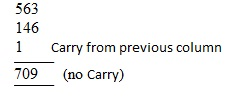Subtraction:

Binary subtraction is done in same way as in decimal system. To subtract binary numbers, First need to explain four simple cases.

Case 1: 0 - 0 = 0

Case 2: 1 - 0 = 1

Case 3: 1 - 1 = 0

Case 4: 10 - 1 = 1

Multiplication and Division:

Multiplication of binary numbers is also done in same manner as in decimal system. It is quite easier, as multiplication table for binary has only four cases.

Case 1: 0x 0 = 0

Case 2: 0x1 = 0

Case 3: 1x0 = 0

Case 4: 1x1 = 1

For example, in multiplying 1101 by 1001, we proceed as follows: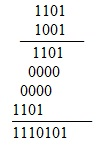In beginning first partial product is written. Then, each partial product is written below previous one by shifting one place towards left relative to previous place. Though, digital circuits or computers add only two binary numbers at a time. Thus, to sum of first two partial products is added third partial product. To this sum is added third partial product to give final sum. Process of dividing binary number is once again the same as followed in decimal system. To divide 1100 by 10, we proceed as follows.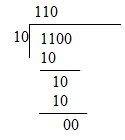Tutorsglobe: A way to secure high grade in your curriculum (Online Tutoring)

Expand your confidence, grow study skills and improve your grades.

Since 2009, Tutorsglobe has proactively helped millions of students to get better grades in school, college or university and score well in competitive tests with live, one-on-one online tutoring.

Using an advanced developed tutoring system providing little or no wait time, the students are connected on-demand with a tutor at www.tutorsglobe.com. Students work one-on-one, in real-time with a tutor, communicating and studying using a virtual whiteboard technology.  Scientific and mathematical notation, symbols, geometric figures, graphing and freehand drawing can be rendered quickly and easily in the advanced whiteboard.

Free to know our price and packages for online physics tutoring. Chat with us or submit request at info@tutorsglobe.com